# Power

Number
${\left(\sqrt{7\cdot \sqrt{7}}\right)}^{9}$
can be written in the form
${7}^{x}$
. Find the value of x.

Correct result:

x =  4.95

#### Solution: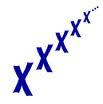We would be pleased if you find an error in the word problem, spelling mistakes, or inaccuracies and send it to us. Thank you!## Next similar math problems:

• Unknown number 7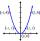Calculate unknown number whose 12th power when divided by the 9th power get a number 27 times greater than the unknown number. Determine the unknown number.
• Exponential equationFind x, if 625 ^ x = 5 The equation is exponential because the unknown is in the exponential power of 625
• Three members GPThe sum of three numbers in GP (geometric progression) is 21 and the sum of their squares is 189. Find the numbers.
• Find the 2Find the term independent of x in the expansion of (4x3+1/2x)8
• 3d vector componentThe vector u = (3.9, u3) and the length of the vector u is 12. What is is u3?
• Circle chordWhat is the length x of the chord circle of diameter 115 m, if the distance from the center circle is 11 m?
• Chord ABWhat is the length of the chord AB if its distance from the center S of the circle k(S, 92 cm) is 10 cm?
• The ticketsThe tickets to the show cost some integer number greater than 1. Also, the sum of the price of the children's and adult tickets, as well as their product, was the power of the prime number. Find all possible ticket prices.
• SquarePoints A[-9,7] and B[-4,-5] are adjacent vertices of the square ABCD. Calculate the area of the square ABCD.
• Cube surface and volumeFind the surface of the cube with a volume of 27 dm3.
• Value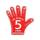Find the value of the expression: 6!·10^-3
• If the 3If the 6th term of a GP is 4 and the 10th is 4/81, find common ratio r.
• BallsThree metal balls with volumes V1=71 cm3 V2=78 cm3 and V3=64 cm3 melted into one ball. Determine it's surface area.
• Unknown number 17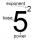Milada said, I am thinking of a number such that I evaluate expression x1/3, the value of the expression would be 5. Which number Milada thinking?
• Exp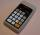If ?, then n is:
• Trapezoid 15Area of trapezoid is 266. What value is x if bases b1 is 2x-3, b2 is 2x+1 and height h is x+4
• A rectangle 2A rectangle has a diagonal length of 74cm. Its side lengths are in ratio 5:3. Find its side lengths.